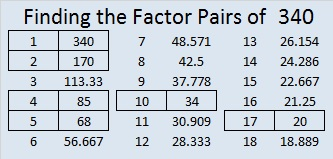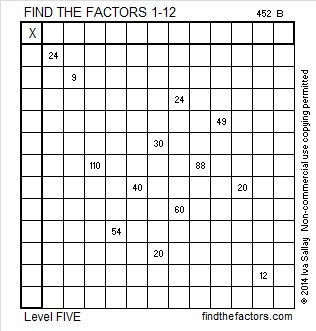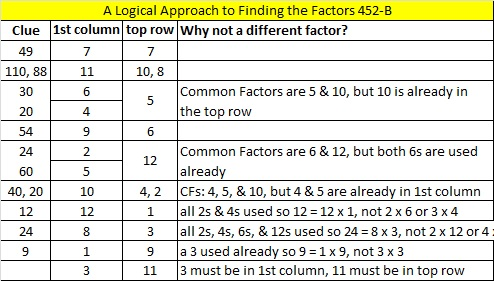# 340 and Level 5

• 340 is a composite number.
• Prime factorization: 340 = 2 x 2 x 5 x 17, which can be written 340 = (2^2) x 5 x 17
• The exponents in the prime factorization are 2, 1, and 1. Adding one to each and multiplying we get (2 + 1)(1 + 1)(1 + 1) = 3 x 2 x 2 = 12. Therefore 340 has exactly 12 factors.
• Factors of 340: 1, 2, 4, 5, 10, 17, 20, 34, 68, 85, 170, 340
• Factor pairs: 340 = 1 x 340, 2 x 170, 4 x 85, 5 x 68, 10 x 34, or 17 x 20
• Taking the factor pair with the largest square number factor, we get √340 = (√4)(√85) = 2√85 ≈ 18.439Do you have any goals to improve your thinking power this year? Well, solving puzzles like this one can be good for your brain!Print the puzzles or type the factors on this excel file: 12 Factors 2014-12-29This site uses Akismet to reduce spam. Learn how your comment data is processed.